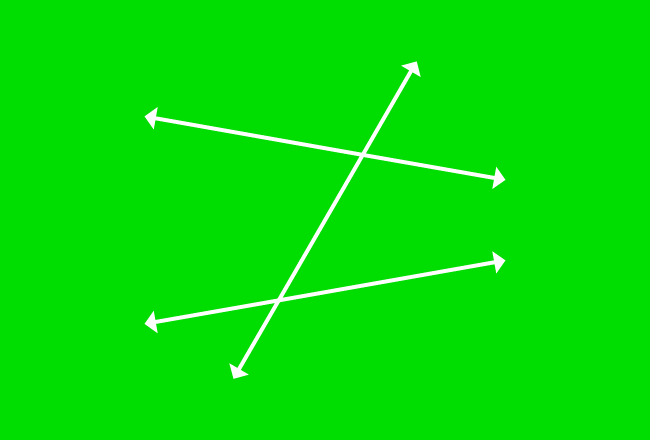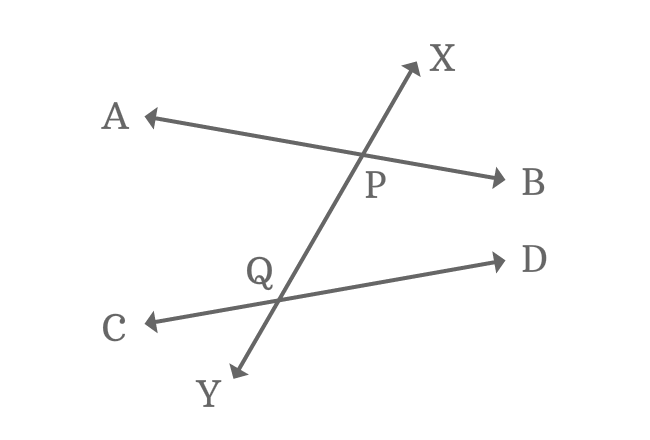# Alternate Exterior angles formed by Nonparallel lines and their TransversalWhen two or more nonparallel lines are intersected by their transversal, the exterior angles which appear opposite sides on the transversal are called exterior alternate angles or alternate exterior angles of nonparallel lines and their transversal.

Total two pairs of exterior angles are formed on opposite sides at the intersection of two nonparallel lines and their transversal. Therefore, the two pairs of exterior angles are called exterior alternate angles or alternate exterior angles.

## Alternate Exterior AnglesThe intersection of two nonparallel lines ($\overleftrightarrow{AB}$ and $\overleftrightarrow{CD}$) by their transversal $\overleftrightarrow{XY}$ formed four exterior angles geometrically known as $\angle APX$, $\angle XPB$, $\angle DQY$ and $\angle YQC$.

$(1) \,\,\,\,\,\,$ $\angle APX$ and $\angle DQY$

$(2) \,\,\,\,\,\,$ $\angle XPB$ and $\angle YQC$

The above two pairs of angles are exterior angles and appear at opposite sides of the intersection of nonparallel lines and their transversal line. Therefore, every pair of exterior angels are called as alternate exterior angles.

### Property

In this case, the alternate exterior angles are not equal because of the involvement of nonparallel lines in the intersection with their transversal.

$(1) \,\,\,\,\,\,$ $\angle APX \ne \angle DQY$

$(2) \,\,\,\,\,\,$ $\angle XPB \ne \angle YQC$

Latest Math Topics
Jun 26, 2023
Jun 23, 2023

Latest Math Problems
Jul 01, 2023
Jun 25, 2023
###### Math Questions

The math problems with solutions to learn how to solve a problem.

Learn solutions

Practice now

###### Math Videos

The math videos tutorials with visual graphics to learn every concept.

Watch now

###### Subscribe us

Get the latest math updates from the Math Doubts by subscribing us.